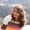FREELessons: 20Length: 1.7 hours• Overview
• Transcript

# 2.6 Imports and Constructors

In this lesson, you'll see how to use functions and classes of other files from main(), by importing packages. You'll also learn how to define constructors in a class header.

## 5.Conclusion1 lesson, 01:17

### 2.6 Imports and Constructors

Hey there, I hope all of you are doing great. In this lesson, we will be learning how do we use classes from different files? This was where we had left in the last lesson. We had this class Geometry defined in the same class in which we have the main function. Now let us create a new file and move this class Geometry, to that file. Moving to the source folder, let us create a new package first. Let's say com.invato. The package name has to be unique for the project. Clicking OK, we have this new package created in our source folder. Here in this package, let us create a new Kotlin class. Because we have the class Geometry, let us give it the name Geometry. Which will be of class type, because we need the Geometry class. Here we have the package as com.envato and the class is Geometry. Let me cut this out and paste it here. And remove the class Geometry from this file. We have an error here. It says, unresolved reference Geometry In this intelligent ID, whenever you get an error like this we can have some suggestions by pressing Alt+Enter. On pressing Alt+Enter we get these options. Import, Create Class, Create Function, Rename Reference. We need to import this class into our file. Since it is in some other package. So we will import the class and here we have the Geometry class imported to our file, import com.envato.Geometry. So this how we work with classes in some other package. We need to import the class, and then instantiate the object of the class. We have the field variable of the Geometry class initialized as element. And then we have called a function display. Let us run the code and see what happens. We get the output here as the element is square. So, we have successfully used the Geometry class in some other package in our main file. Let me show you a small glimpse of how constructor can be defined in Kotlin. So let us define this Geometry class with the constructor here and give the field variable as var figure, which will be of string type. And then remove this figure as the figure variable from the class. Now whatever value for the figure we get while instantiating the object of the geometry will be passed on here. And it has to be of the string type. And then we will use this figure in our functions in the class. Moving to the object instantiation here, we have error. It says no value passed for parameter figure. Since we have figure as a parameter here for the constructor, we need to pass an argument here and we will do it as element. Let us remove it and then call the function display for the Geometry class. Let me change it to circle. We have had enough of squares. Run our code and see what happens. Shift F10. And here we have the element is circle. So we have successfully used the constructor from the class, which is in some other file in our main file. Looking at the syntax again, this is how a constructor is written in Kotlin. We have a parenthesis following the class name and like other programming languages, the constructor are separate functions. Here the constructor Is so concise. We just have parentheses for allowing the class name where we can write the parameters or the arguments that the constructor will have. In case if it didn't have this var figure, which is a string type, it would have been a non parameterized constructor. So this is how concise the code in Kotlin is. So that's all for this lesson. Keep smiling, happy coding and have a good day.

Back to the top